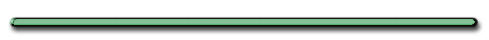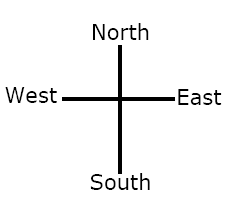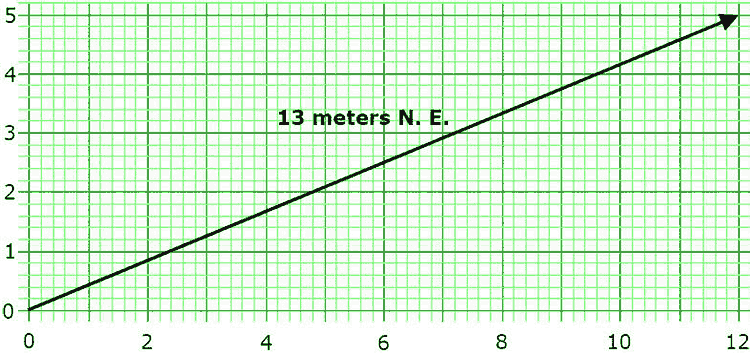AP Physics VelocityA curveball has more than one measureable motion.
How many does it have? (ignore spin)

| Larger |

3 Motions
Forward,
Left (or Right),
Down

Velocity - Speed in a particular direction

| Larger |

C. Velocity - speed in a particular directionVelocity & Direction
St. Mary's H.S.Identify his 2 velocities. Which is the greater of the two?

| Larger |

The answer depends on your coordinate system. The man is moving to the north (or up, forward, y) and the east (or across, to the right, x). Since his easterly displacement is greater, he has a greater easterly velocity

Ex) Joe drove 13 meters North East in 2.0 seconds

What was Joes ...

• Northeast displacement?

• displacement east?

• displacement north?• Northeast displacement?  13 m

• displacement east? 12 m E

• displacement north?  5 m N

Ex) Joe drove 13 meters North East in 2.0 secondsWe can say that Joe has 3 velocities

V = 6.5 m/s N.E.

V = 5 m N/2 seconds =

2.5 m/s NorthV = 12 m East/2seconds

= 6 m/s East

1. Velocity is always written with direction

Examples• 5m/s east

• +5m/s

• 100 m/s downward

• -100 m/s2. Equation:

V = d/t

d = displacement

V = d/t

 Ex) A car travels 90. m due north in 15 secs and  turns around and travels 40. m due south in 5.0 secs. What is the average velocity of the car?

V = (50. m N)/(20. sec)

v = 2.5 m/s NorthAcceleration